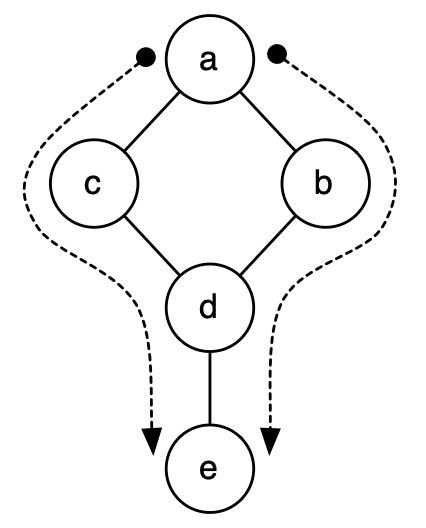시간 제한 메모리 제한 제출 정답 맞은 사람 정답 비율
1 초 128 MB 3 1 1 33.333%

## 문제

On Pandora all Navi are connected by friendships. After carefully mapping friendships between different Navi, Grace wants to measure the strength of the connection between pairs of Navi. She decides the way to calculate this is to treat Navi as nodes in a graph, and friendships between Navi as edges. Then the connection strength between two Navi can be defined as the number different shortest paths each could take to visit the other. Your goal is to help her calculate these values.

Given a list of connections between Navi and two Navi u and v, you must compute the number of different shortest paths between u and v. The length of the path is the number of Navi on the path. Two paths are different if they pass through at least one different Navi.

## 입력

Connections between Navi are described beginning with the line “GRAPH BEGIN”. Additional lines lists individual Navi (nodes), followed (on the same line) by their friends (edges). The line “GRAPH END” ends the list of connection descriptions. The next lines describe pairs of Navi for which answers need to be calculated, each on a single line. Following these lines, a new instance of the problem can be given, starting from scratch.

You may assume all Navi are connected (i.e., any Navi can reach another Navi by some path). Not all Navi will have their connections listed on a separate line: the friendships of some Navi may only be implied by the friendships given on other lines.

## 출력

Your output should consist of pairs of Navi in the same order as in the input, followed by the number of shortest paths between them, both on a single line.

For instance, in the following example the strength of the connection between Navi a and e is 2, since there are 2 paths of length 3 (the shortest possible) from a to e (a → b → d → e and a → c → d → e).

## 예제 입력 1

GRAPH BEGIN
a b c
b d
c d
d e
GRAPH END
a b
a c
a d
a e
b c
b e

## 예제 출력 1

a b 1
a c 1
a d 2
a e 2
b c 2
b e 1


## 힌트